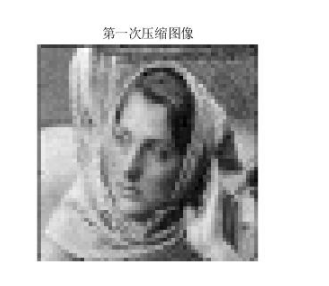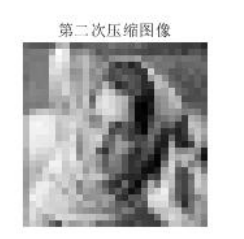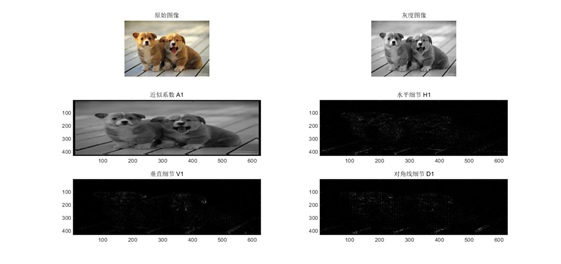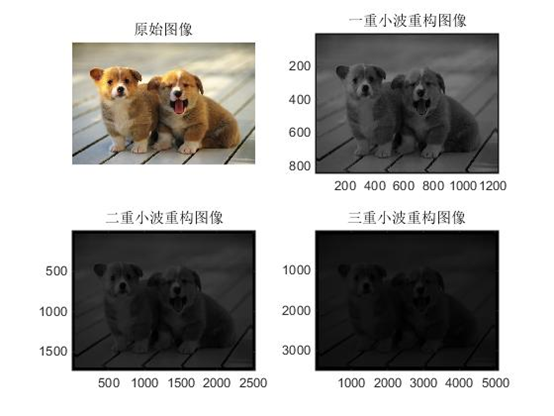2019-10-13 21:00:10 weixin_44225182 阅读数 219
• ###### matlab小波图像处理技术专题

matlab小波图像处理技术专题

339 人正在学习 去看看 刘昱显

## 小波变换实现图像压缩

``````X=imread('a5.jpg');
X=rgb2gray(X);
subplot(221); imshow(X);
title('原始图像');
%对图像用小波进行层小波分解
[c,s]=wavedec2(X,2,'haar');
%提取小波分解结构中的一层的低频系数和高频系数
cal=appcoef2(c,s,'haar',1);
ch1=detcoef2('h',c,s,1);      %水平方向
cv1=detcoef2('v',c,s,1);      %垂直方向
cd1=detcoef2('d',c,s,1);      %斜线方向
%各频率成份重构
a1=wrcoef2('a',c,s,'haar',1);
h1=wrcoef2('h',c,s,'haar',1);
v1=wrcoef2('v',c,s,'haar',1);
d1=wrcoef2('d',c,s,'haar',1);
c1=[a1,h1;v1,d1];
subplot(222),imshow(c1,[]);
title ('分解后低频和高频信息');
%进行图像压缩
%保留小波分解第一层低频信息
%首先对第一层信息进行量化编码
ca1=appcoef2(c,s,'haar',1);
ca1=wcodemat(ca1,440,'mat',0);
%改变图像高度并显示
ca1=0.5*ca1;
subplot(223);imshow(cal,[]);
title('第一次压缩图像');
%保留小波分解第二层低频信息进行压缩
ca2=appcoef2(c,s,'haar',2);
%首先对第二层信息进行量化编码
ca2=wcodemat(ca2,440,'mat',0);
%改变图像高度并显示
ca2=0.25*ca2;
subplot(224);imshow(ca2,[]);
title('第二次压缩图像');
``````2019-08-29 14:35:50 lhw19931201 阅读数 1036
• ###### matlab小波图像处理技术专题

matlab小波图像处理技术专题

339 人正在学习 去看看 刘昱显

``````clear;
X=rgb2gray(A);
%画出原始图像
subplot(2,2,1);imshow(X);
title('原始图像');
%产生含噪图像
x=imnoise(X ,'gaussian',0,0.003);
%画出含噪图像
subplot(2,2,2);imshow(x);
title('含噪声图像');
%下面进行图像的去噪处理
%用小波函数sym4对x进行2层小波分解
[c,s]=wavedec2(x,2,'sym4');
%提取小波分解中第一层的低频图像，即实现了低通滤波去噪
a1=wrcoef2('a',c,s,'sym4');  % a1为 double 型数据；
%画出去噪后的图像
subplot(2,2,3); imshow(uint8(a1)); % 注意 imshow()和image()显示图像有区别，imshow()不能显示 double 型数据，必须进行转换 uint8(a1)；
title('第一次去噪图像');           % 并且image() 显示图像有坐标；
%提取小波分解中第二层的低频图像，即实现了低通滤波去噪
%相当于把第一层的低频图像经过再一次的低频滤波处理
a2=wrcoef2('a',c,s,'sym4',2);
%画出去噪后的图像
subplot(2,2,4); imshow(uint8(a2)); %image(a2);
title('第二次去噪图像');
%保存图像
% imwrite(x,'2.jpg');
% imwrite(uint8(a1),'3.jpg'); %imwrite()保存图像，也需要将数据类型转化为uint8
% imwrite(uint8(a2),'4.jpg');``````2018-08-20 08:25:18 iefenghao 阅读数 12658
• ###### matlab小波图像处理技术专题

matlab小波图像处理技术专题

339 人正在学习 去看看 刘昱显

### 1 小波变换MATLAB程序

``````//小波变换
subplot(2,2,1);
imshow(I);
title('原始图像');
J=rgb2gray(I);
subplot(3,2,2);
imshow(J);
colormap(map);
title('灰度图像');
[cA1,cH1,cV1,cD1] = dwt2(J,'bior3.7');
A1 = upcoef2('a',cA1,'bior3.7',1);
H1 = upcoef2('h',cH1,'bior3.7',1);
V1 = upcoef2('v',cV1,'bior3.7',1);
D1 = upcoef2('d',cD1,'bior3.7',1);
subplot(3,2,3); image(wcodemat(A1,192));
title('近似系数 A1')
subplot(3,2,4); image(wcodemat(H1,192));
title('水平细节 H1')
subplot(3,2,5); image(wcodemat(V1,192));
title('垂直细节 V1')
subplot(3,2,6); image(wcodemat(D1,192));
title('对角线细节 D1')
Y1=idwt2(A1,H1,V1,D1,'bior3.7');
subplot(2,2,2);image(Y1);title('一重小波重构图像');
``````

### 处理之后的图像### 2 多重小波变换MATLAB程序

``````//多重小波变换
subplot(2,2,1);
imshow(I);
title('原始图像');
J=rgb2gray(I);
[cA1,cH1,cV1,cD1] = dwt2(J,'bior3.7');
A1 = upcoef2('a',cA1,'bior3.7',1);
H1 = upcoef2('h',cH1,'bior3.7',1);
V1 = upcoef2('v',cV1,'bior3.7',1);
D1 = upcoef2('d',cD1,'bior3.7',1);
Y=idwt2(A1,H1,V1,D1,'bior3.7');
subplot(2,2,2);image(Y);title('一重小波重构图像');
A1 = upcoef2('a',cA1,'bior3.7',2);
H1 = upcoef2('h',cH1,'bior3.7',2);
V1 = upcoef2('v',cV1,'bior3.7',2);
D1 = upcoef2('d',cD1,'bior3.7',2);
Y=idwt2(A1,H1,V1,D1,'bior3.7');
subplot(2,2,3);image(Y);title('二重小波重构图像');
A1 = upcoef2('a',cA1,'bior3.7',3);
H1 = upcoef2('h',cH1,'bior3.7',3);
V1 = upcoef2('v',cV1,'bior3.7',3);
D1 = upcoef2('d',cD1,'bior3.7',3);
Y=idwt2(A1,H1,V1,D1,'bior3.7');
subplot(2,2,4);image(Y);title('三重小波重构图像');
``````

### 实验结果• ###### matlab小波图像处理技术专题

matlab小波图像处理技术专题

339 人正在学习 去看看 刘昱显

http://media.cs.tsinghua.edu.cn/~ahz/digitalimageprocess/chapter12/chapt12_ahz.htm

Matlab 小波变换

lean图像的行列应该满足2的幂次方

```img  =  imread('lena.jpg');
img =rgb2gray(img);
img = double(img);

[ca1 ch1,cv1,cd1] = dwt2(img,'haar');
figure;
imshow([uint8(ca1),uint8(ch1);uint8(cv1),uint8(cd1)]);
title('first wavelet decomposition');

[ca2 ch2,cv2,cd2] = dwt2(ca1,'haar');
figure;
imshow([uint8(ca2),uint8(ch2);uint8(cv2),uint8(cd2)]);
title('second wavelet decomposition');

% restruct img
img_ca1 =  idwt2(ca2,ch2,cv2,cd2,'haar');
img_re = idwt2(img_ca1,ch1,cv1,cd1,'haar');
figure;
imshow( uint8(img_re));
title('wavelet restruct');```

2015-10-27 18:13:44 robin1987z 阅读数 11660
• ###### matlab小波图像处理技术专题

matlab小波图像处理技术专题

339 人正在学习 去看看 刘昱显
##### 小波变换原理

所谓的小波的小是针对傅里叶波而言，傅里叶波指的是在时域空间无穷震荡的正弦（或余弦波）。

相对而言，小波指的是一种能量在时域非常集中的波，它的能量有限，都集中在某一点附近，而且积分的值为零，这说明它与傅里叶波一样是正交波。

举一些小波的例子：众所周知，图像的傅里叶变换是将图像信号分解为各种不同频率的正弦波。同样，小波变换是将图像信号分解为由原始小波位移和缩放之后的一组小波。

小波在图像处理里被称为图像显微镜，原因在于它的多分辨率分解能力可以将图片信息一层一层分解剥离开来。剥离的手段就是通过低通和高通滤波器，

1.用低通滤波器与原始像素矩阵做卷积得：[8 10 12 15 12 14 12 5]/sqrt(2)

2.用高通滤波器与原始像素矩阵做卷积得：[-4 2 -4 1 2 -4 6 1]/sqrt(2)

1.对L数组插值得：[0 10 0 15 0 14 0 5]/sqrt(2)

2.对H数组插值得：[0 2 0 1 0 -4 0 1]/sqrt(2)

##### 基于小波变换的图像压缩